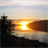# App Development

Discussion board where members can learn more about Qlik Sense App Development and Usage.

Announcements
April 22, 2PM EST: Learn about GeoOperations in Qlik Sense SaaS READ MORE
cancel
Showing results for
Search instead for
Did you mean:Creator II

## Set anaylsis expression is not working

I am using below expression in a text box, and it's just giving me blank values:-

=sum({<[YTD-flag]={1},[XYZName]={'\$(=\$(=vUserXYZ))'}>}[abc_\$(vCurrency_Fxx)])

Please suggest, what is causing an error

Labels (1)
• ### Set Analysis

1 Solution

Accepted SolutionsPartner

then [XYZName]={'\$(vUserXYZ)'} should be enough i believe. try that .

For simple variables the way you defined it should work \$(=\$(=vUserXYZ)). i am just wondering if the complexity of the variable and the double \$ sign expansion somehow messes things up

8 RepliesPartner

How is your variable defined?Partner

i would try and define the variable with abc inside it?

i.e. instead of [abc_\$(vCurrency_Fxx)]) just use \$(vCurrency_Fxx_dim)

where vCurrency_Fxx_dim is 'abc_' &\$(vCurrency_Fxx)Creator II
Author

=sum({<[YTD-flag]={1},[XYZName]={'\$(=\$(=vUserXYZ))'}>}[abc_\$(vCurrency_Fxx)])

In above expression, [abc_\$(vCurrency_Fxx)], this variable is working fine, if I just do sum([abc_\$(vCurrency_Fxx)]), I am getting values.

Issue is with [XYZName]={'\$(=\$(=vUserXYZ))'}

where vUserXYZ=if(GetSelectedCount([XYZ Name]) = 1,[XYZName],If(IsNull(Only({<[User]={'\$(=\$(vUser))'}>} Distinct [User APH])), 'Product Hierarchy structure'
,Only({<[User]={'\$(=\$(vUser))'}>} Distinct [User APH]))
)Partner

do you have an = in from of the expression in vUserXYZ?Creator II
Author

Yes, Variable expression is define with an =Partner

then [XYZName]={'\$(vUserXYZ)'} should be enough i believe. try that .

For simple variables the way you defined it should work \$(=\$(=vUserXYZ)). i am just wondering if the complexity of the variable and the double \$ sign expansion somehow messes things upCreator II
Author

What if I won't use an = sign in variable expression, in that case it should work?

[XYZName]={\$(=\$(=vUserXYZ))}

But even after using it, not getting proper value.Partner

it should. it does for simpler test i did